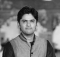## JavaScript Program to Find difference between sums of two diagonalsAmitDiwan

Updated on 15-Mar-2023 14:31:38

We will be finding the difference between the sums of two diagonals of a square matrix. Firstly, we will calculate the sum of the elements present in the first diagonal by traversing the matrix starting from the top left corner to the bottom right corner. Secondly, we will calculate the ... Read More

## JavaScript Program to Find closest number in arrayAmitDiwan

Updated on 15-Mar-2023 14:30:35

We will write a JavaScript program to find the closest number in an array by comparing each element with the target number and keeping track of the closest one. The program will use a loop to go through each element in the array and use a conditional statement to compare ... Read More

## JavaScript Program to Find array sum using Bitwise OR after splitting given array in two halves after K circular shiftsAmitDiwan

Updated on 15-Mar-2023 14:29:03

We will write a JavaScript program to find the sum of an array by using bitwise OR after splitting the given array into two halves after K circular shifts. Our program will perform the task by taking an array and an integer K as inputs. First, we will split the ... Read More

## JavaScript program to find area of a circleAmitDiwan

Updated on 13-Mar-2023 16:55:48

We will be using the below formula to find the area of a circle − pi * r^2 In the program, we will prompt the user to input the radius of the circle. Then, we will use the formula to calculate the area and print it on the ... Read More

## JavaScript program to find Area and Perimeter of RectangleAmitDiwan

Updated on 13-Mar-2023 16:54:09

We are writing a JavaScript program to calculate the area and perimeter of a rectangle. The program will prompt the user to input the width and length of the rectangle, and then we will use these values to calculate the area and perimeter. We will be continuously using these formulas: ... Read More

## JavaScript Program to Find a triplet that sum to a given valueAmitDiwan

Updated on 13-Mar-2023 16:51:34

We are going to write a JavaScript program to find a triplet that sum up to a given value. This program will make use of nested loops to iterate over the input array and check for the existence of a triplet with a sum equal to the given value. Our ... Read More

## JavaScript Program to Find a triplet such that sum of two equals to third elementAmitDiwan

Updated on 13-Mar-2023 16:47:59

We will be writing a JavaScript program that finds a triplet where the sum of two elements equals the third element. This program will be implemented using arrays and loop structures. We will be iterating through the array and checking for each element if the sum of two elements equal ... Read More

## JavaScript Program to Efficiently compute sums of diagonals of a matrixAmitDiwan

Updated on 13-Mar-2023 16:44:30

We are going to write a program in JavaScript that efficiently computes the sum of the diagonals of a matrix. To do this, we will utilize a loop structure that iterates through the matrix and adds the elements located at the positions that correspond to the diagonals. By taking advantage ... Read More

## JavaScript Program To Delete Nodes Which Have A Greater Value On Right SideAmitDiwan

Updated on 13-Mar-2023 16:42:33

We will be implementing a function to delete nodes in a linked list which have a greater value on their right side. The approach is to traverse the linked list from right to left and keep track of the maximum value encountered so far. For each node, we will compare ... Read More

## JavaScript Program To Delete Alternate Nodes Of A Linked ListAmitDiwan

Updated on 13-Mar-2023 16:34:29

We will be writing a JavaScript program to delete alternate nodes of a linked list. We will be utilizing a while loop to traverse the linked list while keeping a track of the current and previous node. In each iteration of the loop, we will be skipping the current node ... Read More